## Rotation Matrix

When discussing a Rotation, there are two possible conventions: rotation of the axes and rotation of the object relative to fixed axes.In, let a curve be rotated by a clockwise Angle, so that the original axes of the curve areand, and the new axes of the curve are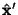and. The Matrix transforming the original curve to the rotated curve, referred to the originalandaxes, is(1)

i.e.,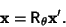(2)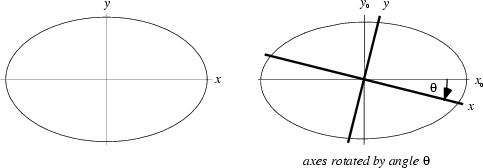On the other hand, let the axes with respect to which a curve is measured be rotated by a clockwise Angle, so that the original axes areand, and the new axes areand. Then the Matrix transforming the coordinates of the curve with respect toandis given by the Matrix Transpose of the above matrix:(3)

i.e.,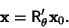(4)

In, rotations of the-,-, and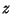-axes give the matrices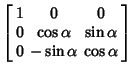(5)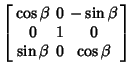(6)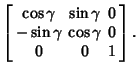(7)